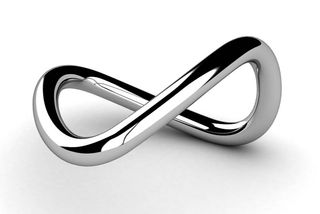# No End in Sight: Debating the Existence of InfinityThe mathematical symbol that represents infinity.

NEW YORK — Despite being in existence for more than 2,000 years, the concept of infinity has endured as an enigmatic, and oftentimes challenging, idea for mathematicians, physicists and philosophers. Does infinity really exist, or is it just part of the fabric of our imaginations?

A panel of scientists and mathematicians gathered to discuss some of the profound questions and controversies surrounding the concept of infinity here Friday (May 31), as part of the World Science Festival, an annual celebration and exploration of science.

Part of the difficulty in trying to solve some of the abstract questions related to infinity is that these problems fall beyond the more established mathematical theories, said William Hugh Woodin, a mathematician at the University of California, Berkeley. [Watch: World Science Festival Highlights]

"It's kind of like mathematics lives on a stable island — we've built them a solid foundation," Woodin said. "Then, there's the wild land out there. That's infinity."

Where it all began

A philosopher named Zeno of Elea, who lived from 490 B.C. to 430 B.C, is credited with introducing the idea of infinity.

The concept was studied by ancient philosophers, including Aristotle, who questioned whether infinites could exist in a seemingly finite physical world, said Philip Clayton, dean of the Claremont School of Theology at Claremont Lincoln University in Claremont, Calif. Theologians, including Thomas Aquinas, used the infinite to explain the relationship between humans, God and the natural world.

In the 1870s, a German mathematician named Georg Cantor pioneered work in a field that became known as set theory. According to set theory, integers, which are numbers without a fraction or decimal component (such as 1, 5, -4), make up an infinite set that is countable. On the other hand, real numbers, which include integers, fractions and so-called irrational numbers, such as the square root of 2, are part of an infinite set that is uncountable.

This led Cantor to wonder about different types of infinity.

"If there are now two kinds of infinity — the countable kind and this continuous kind, which is bigger — are there other infinities? Is there some infinity that's sandwiched in between them?" said Steven Strogatz, a mathematician at Cornell University in Ithaca, N.Y.

Cantor believed that no infinities exist between the sets of integers and real numbers, but he was never able to prove it. His statement, however, became known as the continuum hypothesis, and mathematicians who tackled the problem in Cantor's footsteps were labeled set theorists.

Exploring beyond

Woodin is a set theorist, and has spent his life trying to solve the continuum hypothesis. To date, mathematicians have not been able to prove or disprove Cantor's postulation. Part of the problem is that the idea that there are more than two types of infinity is so abstract, Woodin said.

"There's no satellite you can build to go out and measure the continuum hypothesis," he explained. "There's nothing in our world around us that will help us determine whether or not the continuum hypothesis is true or false, as far as we know." [5 Seriously Mind-Boggling Math Facts]

Trickier still is the fact that some mathematicians have dismissed the relevance of this type of mathematical work.

"These people in set theory strike us, even in math, as sort of strange," Strogatz joked. But, he said he understands the importance of the work being done by set theorists, because if the continuum hypothesis is proven false, it could uproot basic mathematical principles in the same way that contradicting number theory would wipe out the bases for math and physics.

"We know that they're doing really deep, important work, and in principle, it's foundational work," Strogatz explained. "They're shaking the foundations that we're all working on, up on the second and third floors. If they mess something up, it could tip us all over."

The future of mathematics

Still, despite all of the uncertainties, the work done by set theorists could have positive ripple effects that serve to strengthen the foundations of mathematics, Woodin said.

"By investigating infinity, and to the extent that we can be successful, I think we make the case for the consistency of arithmetic," he explained. "That's a bit of a fanatical statement, but if infinity doesn't lead to a contradiction, certainly the finite doesn't lead to a contradiction. So, maybe by exploring the outer reaches to see if there is a contradiction, you gain some security."

The paradoxes that characterize the concept of infinity are perhaps best explained with the number pi, Strogatz said. Pi, one of the most recognizable mathematical constants, represents the ratio of a circle's circumference to its diameter. Among its myriad applications, pi can be used to find the area of a circle.

"Pi is typical of real numbers … in that it has this infinite amount of unpredictable information in it, and at the same time, is so totally predictable," Strogatz said. "There's nothing more orderly than a circle, which pi embodies — it's the very symbol of order and perfection. So this coexistence of perfect predictability and order, with this tantalizing mystery of infinite enigma built into the same object, is part of the pleasure of our subject and, I suppose, of infinity itself."